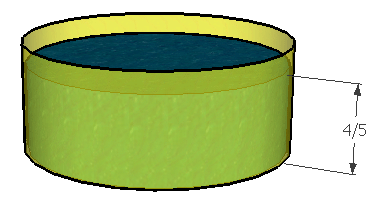SEARCH HOMEMath Central Quandaries & QueriesQuestion from nadha, a student: A water pump can fill a tank at the rate of 750 litres per hour. A tank has a capacity of 9000 litres.Find the time needed to fill 7/15 of the tank? I find difficult to do this question pls explain this with full method, pls explain me with full method.I'll show you how to solve this kind of question and you can use this method for your question.

My garden hose can deliver water at the rate of 2 000 liters per hour. My pool has a capacity of 15 000 liters. How long will it take my to fill it to 4/5 capacity?First, I can find out how much water I need. Since I am filling the tank to the 4/5ths level, I need 4/5 of the total capacity of my pool (15 000) liters. So I need 4/5 x 15 000, which is 12 000 liters.

Then I find out how long I need to run the water to get this much water. Remember that time x rate = amount. So that is the same as saying that amount divided by rate equals time! (for example, 220 km divided by 110 km/hr equals 2 hours of driving time)

So 12 000 / 2 000 = 6 hours.

Now you try your question. First find the amount of water you need, then use that to find out the time you need.

Cheers,
Stephen La RocqueMath Central is supported by the University of Regina and The Pacific Institute for the Mathematical Sciences.